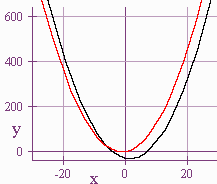$$\newcommand{\id}{\mathrm{id}}$$ $$\newcommand{\Span}{\mathrm{span}}$$ $$\newcommand{\kernel}{\mathrm{null}\,}$$ $$\newcommand{\range}{\mathrm{range}\,}$$ $$\newcommand{\RealPart}{\mathrm{Re}}$$ $$\newcommand{\ImaginaryPart}{\mathrm{Im}}$$ $$\newcommand{\Argument}{\mathrm{Arg}}$$ $$\newcommand{\norm}{\| #1 \|}$$ $$\newcommand{\inner}{\langle #1, #2 \rangle}$$ $$\newcommand{\Span}{\mathrm{span}}$$

# 2.3: Curve Intersection

$$\newcommand{\vecs}{\overset { \rightharpoonup} {\mathbf{#1}} }$$ $$\newcommand{\vecd}{\overset{-\!-\!\rightharpoonup}{\vphantom{a}\smash {#1}}}$$$$\newcommand{\id}{\mathrm{id}}$$ $$\newcommand{\Span}{\mathrm{span}}$$ $$\newcommand{\kernel}{\mathrm{null}\,}$$ $$\newcommand{\range}{\mathrm{range}\,}$$ $$\newcommand{\RealPart}{\mathrm{Re}}$$ $$\newcommand{\ImaginaryPart}{\mathrm{Im}}$$ $$\newcommand{\Argument}{\mathrm{Arg}}$$ $$\newcommand{\norm}{\| #1 \|}$$ $$\newcommand{\inner}{\langle #1, #2 \rangle}$$ $$\newcommand{\Span}{\mathrm{span}}$$ $$\newcommand{\id}{\mathrm{id}}$$ $$\newcommand{\Span}{\mathrm{span}}$$ $$\newcommand{\kernel}{\mathrm{null}\,}$$ $$\newcommand{\range}{\mathrm{range}\,}$$ $$\newcommand{\RealPart}{\mathrm{Re}}$$ $$\newcommand{\ImaginaryPart}{\mathrm{Im}}$$ $$\newcommand{\Argument}{\mathrm{Arg}}$$ $$\newcommand{\norm}{\| #1 \|}$$ $$\newcommand{\inner}{\langle #1, #2 \rangle}$$ $$\newcommand{\Span}{\mathrm{span}}$$

## Intersection of Lines

Recall that if we want to find the intersection point of two lines, we have two choices: substitution and elimination.

Example 1: Substitution

Solve

$x + 2y = 5,$

$4x - 3y = -2.$

Solutions

We use the first equation to solve for $$x$$:

$x = 5 - 2y$

then we plug this into the second equation to get

\begin{align} 4(5 - 2y) - 3y &= -2 \\ -11y + 20 &= -2 \\ y &= 2 \end{align}

and stick this back into the equation for $$x$$ to get:

$x = 5 - 2(2) = 1.$

Example 2: Elimination

Solve

$2x + 5y = 19$

$3x - 5y = -9.$

We add the equation to get

$5x = 10,\; x = 2$

Hence

$2(2) + 5y = 19$

$y = 3.$

## Intersection of Other Curves

Example 3: Substitution

Find the intersection of the curves

$x^2 + y^2 = 25$

and

$y = \dfrac{1}{3}x + 3.$We use the method of substitution to arrive at

\begin{align} x^2+\left(\dfrac{1}{3}x+3\right)^2&=25 \\ x^2+\dfrac{1}{9}x^2+2x+9&=25 \\ 10x^2+18x-144&=0 \\ 5x^2+9x-72&=0 \\ (5x+24)(x-3)&=0 \end{align}

$x=-\dfrac{24}{5} \; \text{ or }\; x=3$

$y=(\dfrac{1}{3}) \left(\dfrac{-24}{5}\right)+3 \;\;\; \text{or} \;\;\; y=\dfrac{1}{3}(3)+3$

$y=-\dfrac{7}{5} \; \text{ or } \; y=4.$

We get the points

$$\left(-\dfrac{24}{5},-\dfrac{7}{5}\right)$$ and $$(3,4)$$.

Example 4: Elimination

$x^2 + 2y^2 = 18$

$2x^2 + y^2 = 15$We multiply the first equation by 2 and subtract the second equation to get:

\begin{align} 3y^2&= 21 \\ y^2&= 7 \\ y &= \sqrt{7} \;\;\; \text{or} \;\;\; y = -\sqrt{7} \end{align}

Substituting back into the first equation, we get:

$x^2 + 2(7) = 18$

$x = 2 \;\;\; \text{or} \;\;\; x = -2,$

hence we get the four points:

$(2,\sqrt{7}), (-2,\sqrt{7}), (2,-\sqrt{7}), (-2,-\sqrt{7}).$

Example 5: Using a Graphing Calculator

We will use a graphing calculator to find the intersection of

$y^2+ 16x = 0$

and

$y^2+ 9x^2-18x = 18.$To find the intersection we just use the intersection function on the graphing calculator.

Example 6

We will use the intercept method to solve

$(x - 7)(x + 4) = (x + 1)^2.$

We find the intersection of the two curves

$y = (x - 7)(x + 4)$

and

$y = (x + 1)^2.$Larry Green (Lake Tahoe Community College)